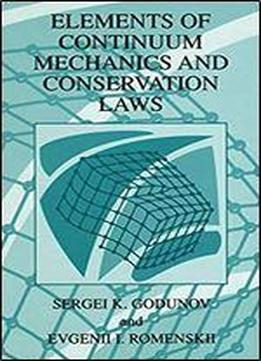# Elements Of Continuum Mechanics And Conservation Laws by S.K. Godunov / 2003 / English / PDF

Elements of Continuum Mechanics and Conservation Laws presents a systematization of different models in mathematical physics, a study of the structure of conservation laws, thermodynamical identities, and connection with criteria for well-posedness of the corresponding mathematical problems. The theory presented in this book stems from research carried out by the authors concerning the formulations of differential equations describing explosive deformations of metals. In such processes, elasticity equations are used in some zones, whereas hydrodynamics equations are stated in other zones. Plastic deformations appear in transition zones, which leads to residual stresses. The suggested model contains some relaxation terms which simulate these plastic deformations. Certain laws of thermodynamics are used in order to describe and study differential equations simulating the physical processes. This leads to the special formulation of differential equations using generalized thermodynamical potentials.

views: 444